# AP Calculus BC Practice Test 34

### Test Information9 questions27 minutes

Calculator Allowed

1. Two objects in motion from t = 0 to t = 3 seconds have positions x1(t) = cos (t2 + 1) and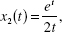respectively. How many times during the 3 seconds do the objects have the same velocity?

2. The table below shows values of f (x) for various values of x:

 x -1 0 1 2 3 f ″(x) -4 -1 2 5 8

The function f could be

3. Where, in the first quadrant, does the rose r = sin 3θ have a vertical tangent?

4. A cup of coffee placed on a table cools at a rate of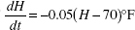per minute, where H represents the temperature of the coffee and t is time in minutes. If the coffee was at 120° F initially, what will its temperaturebe 10 minutes later?

5. An investment of \$4000 grows at the rate of 320e0.08t dollars per year after t years. Its value after 10 years is approximately

6.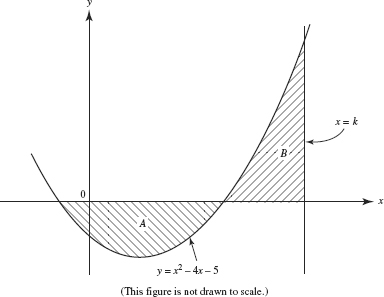The sketch shows the graphs of f (x) = x2 - 4x - 5 and the line x = k. The regions labeled A and B have equal areas if k =

7. The graph below shows the velocity of an object during the interval 0 ≤ t ≤ 9.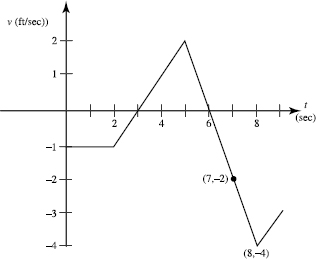The object attains its greatest speed at t =

8. The graph below shows the velocity of an object during the interval 0 ≤ t ≤ 9.The object was at the origin at t = 3. It returned to the origin

9. An object in motion in the plane has acceleration vector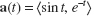for 0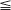t5. It is at rest when t = 0. What is the maximum speed it attains?